# Counters, Accumulators,and Comparing Loop TypesThis activity extends the “Teaching Foundations of Computer Science With Python on TI-Nspire™ Technology” workshop to study additional topics from computer science with the support of the TI-Nspire™ CX II graphing calculator with Python coding technology. We will compare and contrast the three types of loops explored in this workshop (For, While, and Do While), then we will explore counters and accumulators and apply our learning about loops.

In this activity, we will:

• Compare and contrast pre-test (For, While) and post-test (Do While) loops
• Use counter and accumulator statements
• Use loop control structures and conditional statements in a program to get an undetermined amount of data

## Materials for learning

Also, you’ll want to have the following handy:

• TI-Nspire™ CX II graphing calculator (with updated OS)

## Comparing For, While and Do While loops

How do the three loop structures prevalent in computer science languages (For, While, and Do While loops) compare to and contrast with each other? What are the implications of these similarities and differences?

Through the last three activities in the workshop, we have explored each loop structure in isolation. Now, we will step back and consider these three iterative control structures in relation to each other. How are they similar? How are they different?

### Comparing and contrasting loop structures

Consider the three programs shown in the images below. These three programs are designed to model a For loop, a While loop and a Do While loop respectively.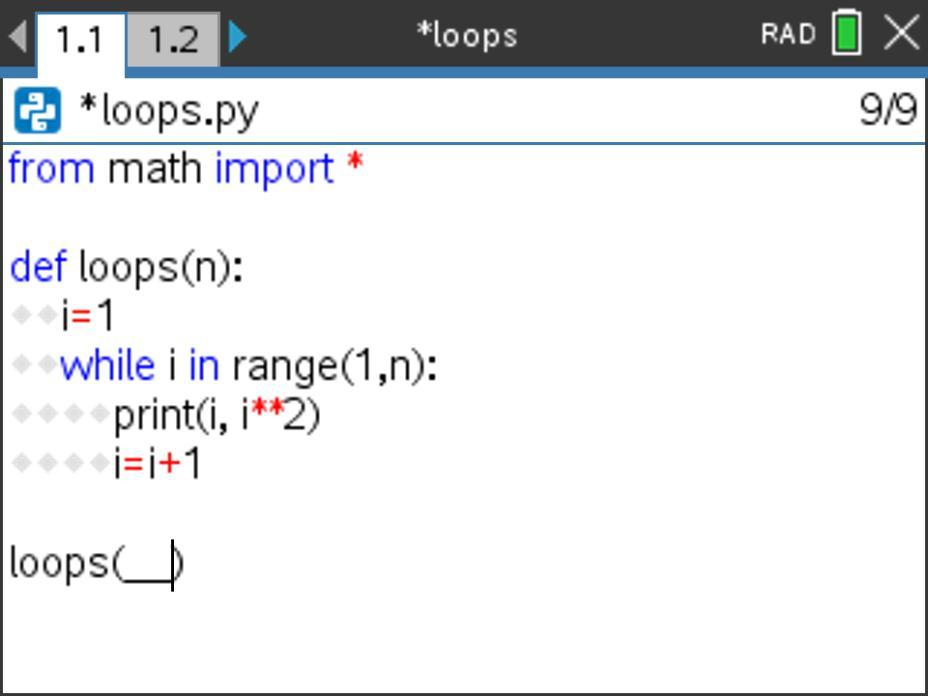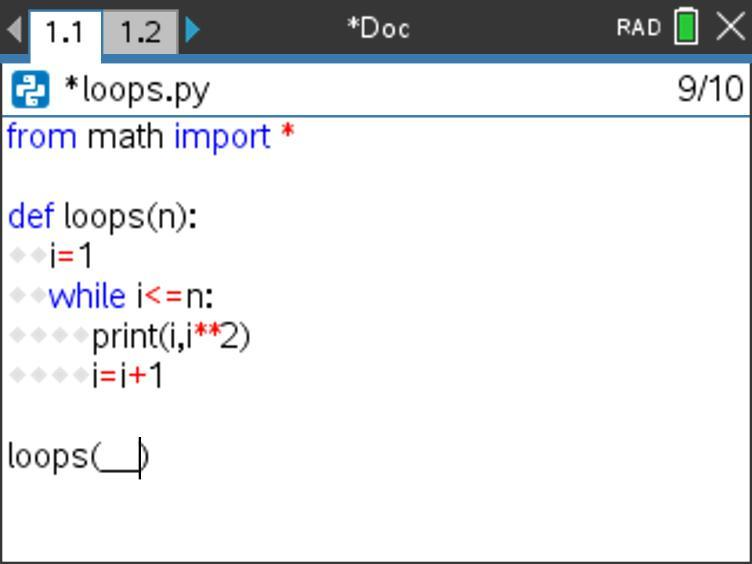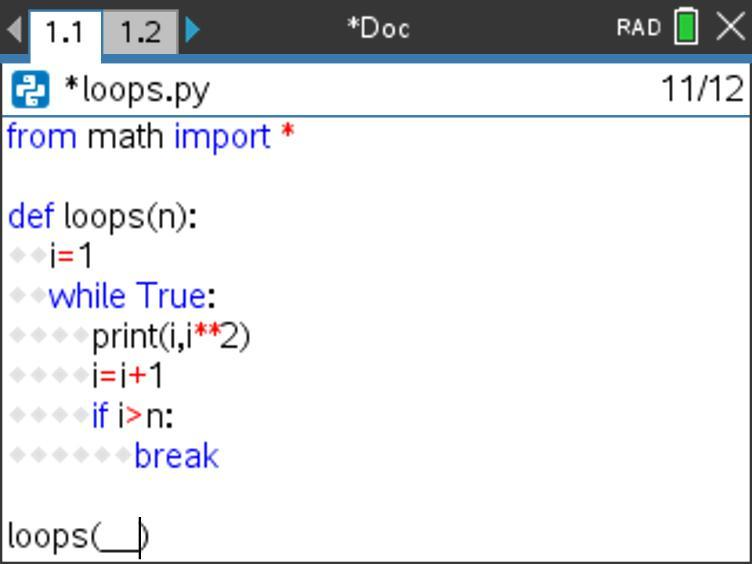What similarities do you notice across these programs?

We might notice that each program uses a formal parameter of n and a local loop variable of i. Each loop includes the same action within it of print(i,i2). (Recall that i**2 is Python for i2 and that you need to include from math import * to use this command.)

What other similarities do you notice? What differences do you notice across these programs?

We might notice that the loop variable i is explicitly initialized before the While and Do While loops, but that this initialization is included in the For loop statement.

We might also notice that the loop variable i is explicitly incremented within the body of each of the loops (with statements of i=i+1). (Note that here we are using a While loop to model a For loop and another While loop to model a Do While loop. Why?)

We might notice that the programs check similar conditions but in different ways: the For loop increments from 1 to n; the While loop checks to see if i n (in order to decide if the loop should run again); and, the Do While loop checks to see if i > n (in order to decide if the loop should break and not run again).

Which of these differences are superficial, and which differences are substantive? What are the ramifications of these differences?

### Analyzing loop outputs

Imagine that we executed each of the three programs shown above with a method call of loops(2). What do you think the output of each loop would be? How do you know? Take a minute to predict the output for each version of the loops() program if it was called with a method call of loops(2).

Here is a screenshot showing these outputs. How do these results compare to your predictions?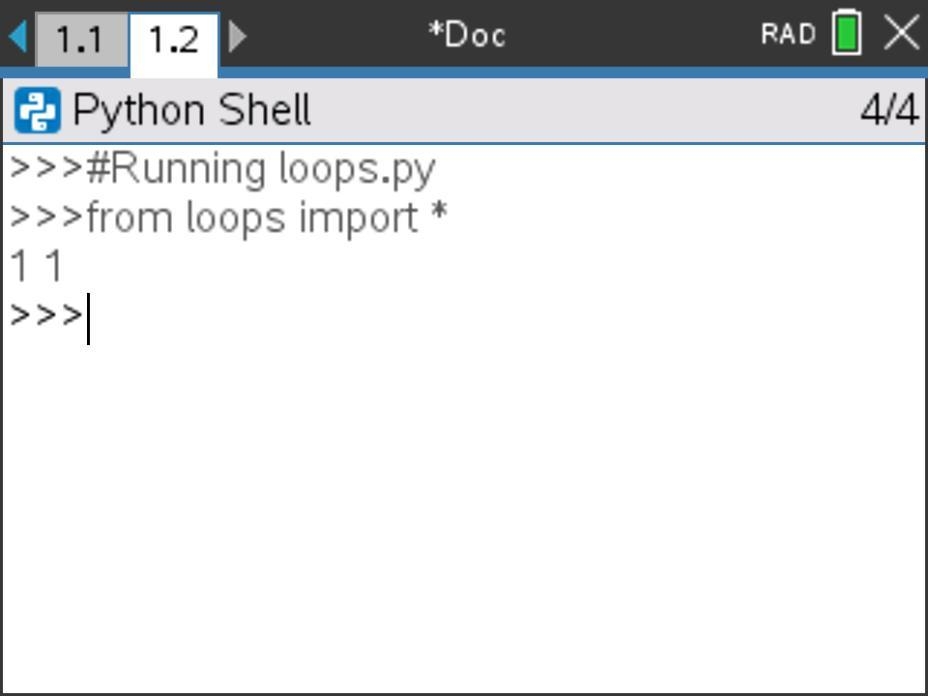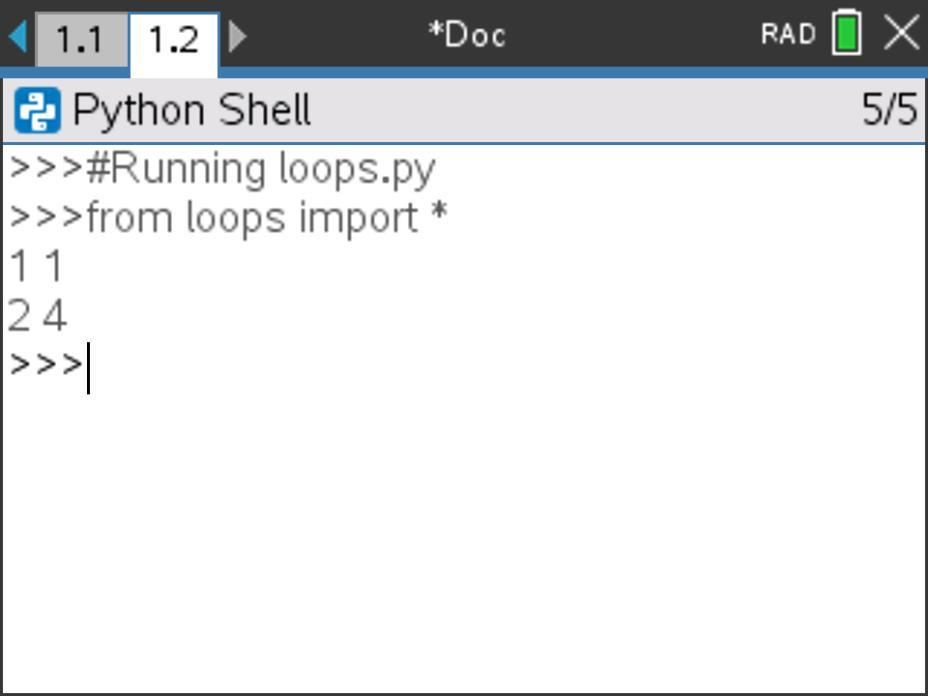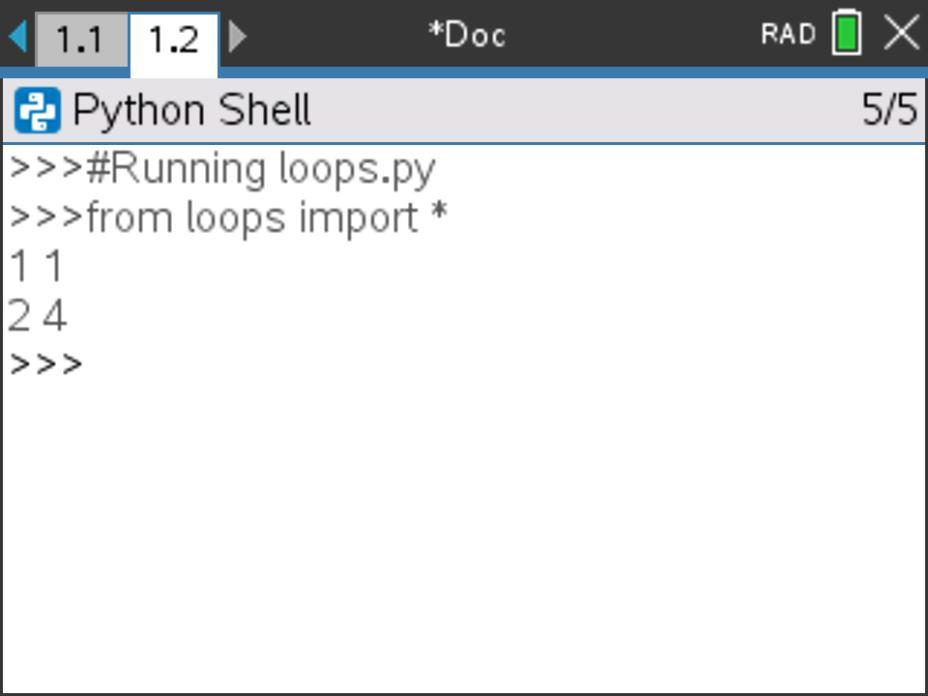Interesting. So, two of the programs produced the same output for the method call of loops(2). Are these programs somehow equivalent? Despite their seemingly cosmetic differences, do these programs actually output the same results all the time? In other words, will the output of these three different programs always be the same? What might be a boundary condition that would be productive to test in this instance?

But, wait — why didn’t the For loop produce the same output? This is because the range(a,b) command doesn’t include the value of b. Specifically, in this case, range(1,n) where n = 2 will only include the value 1. To make it include 1 and 2, change the code to while i in range(1,n+1).

Imagine that we executed each program with a method call of loops(0). What do you think the output of each loop would be? How do you know? Take a minute to predict the output for each version of the loops() program if it was called with a method call of loops(0).

Here is a screenshot showing these outputs. How do these results compare to your predictions?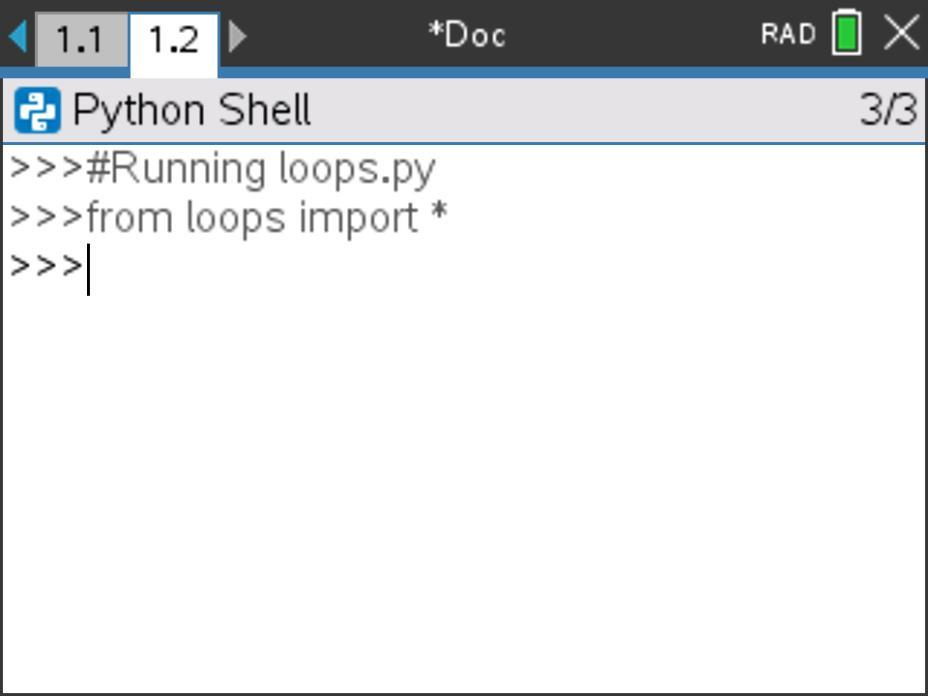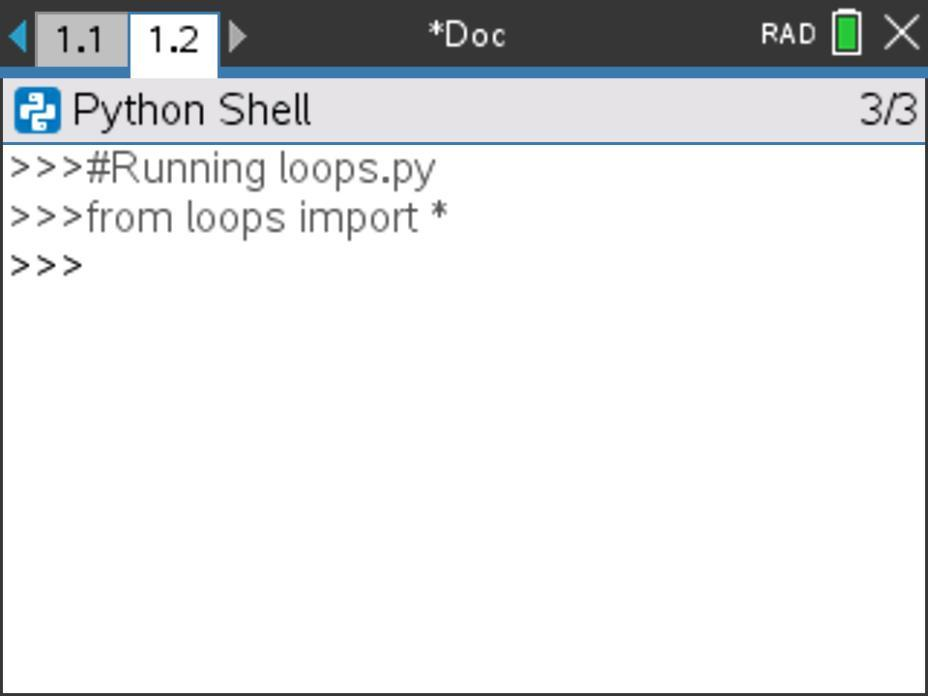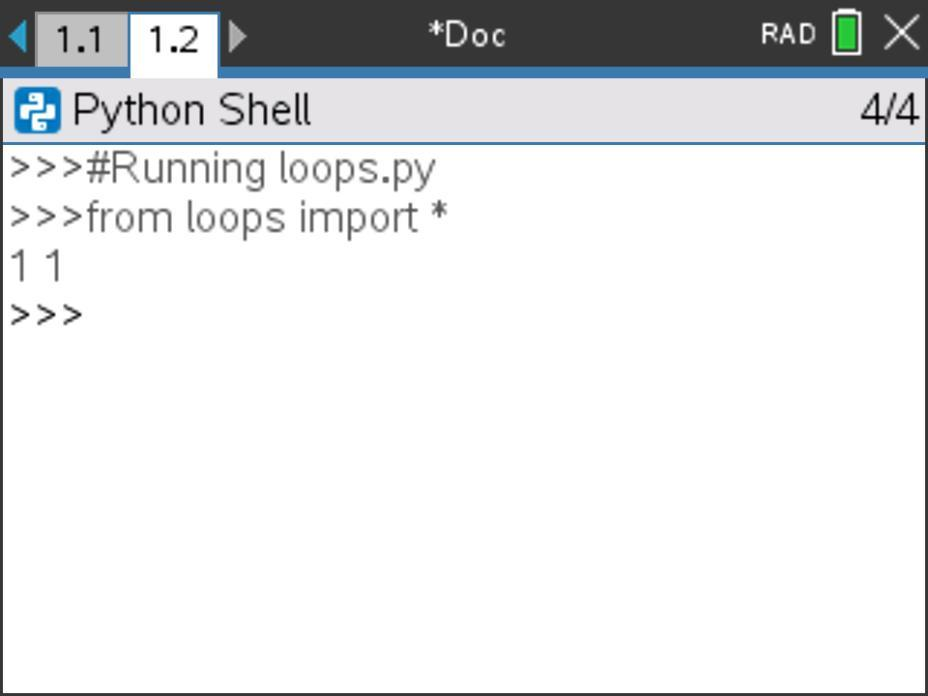These results are interesting again. Why does the Do While loop produce an output for loops(0) while the For loop and While loop do not?

The two pre-test loops (For and While) check the condition (“Is in?”, or, specifically, in this case “Is 1 ≤ 0?”) before the loop is entered. As such, the check determines that an input of 0 makes this initial condition false, so neither loop will run even once. The post-test loop (Do While) doesn’t check the condition until it has run at least once. So, even though the initial value of 0 doesn’t make the condition true, this isn’t checked until the end of the loop, so the loop has already run once. This illustrates a really important idea about loops — specifically, which types of loops might never run (pre-test loops with a false initial condition) and which types of loops will always run at least once (post-test loops regardless of the truth value of the initial condition).

How might we determine a boundary condition that would be productive to test in order to determine whether these loops produce different outputs in some instances? Consider an initial value for the actual parameter that would make the condition for the pre-test loops false, then execute all three loops using this actual parameter. This is what we did when we considered the output of each loop for a method call of loops(0).

### Formalizing the three types of loops

Let’s revisit the Loops notes page used in the workshop to formalize the three types of loops we have explored in this activity.

Here are two pictures of the notes page where we can capture our important learnings about For, While and Do While loops. The interactive notes page uses the three versions of the loops() program that we traced through above.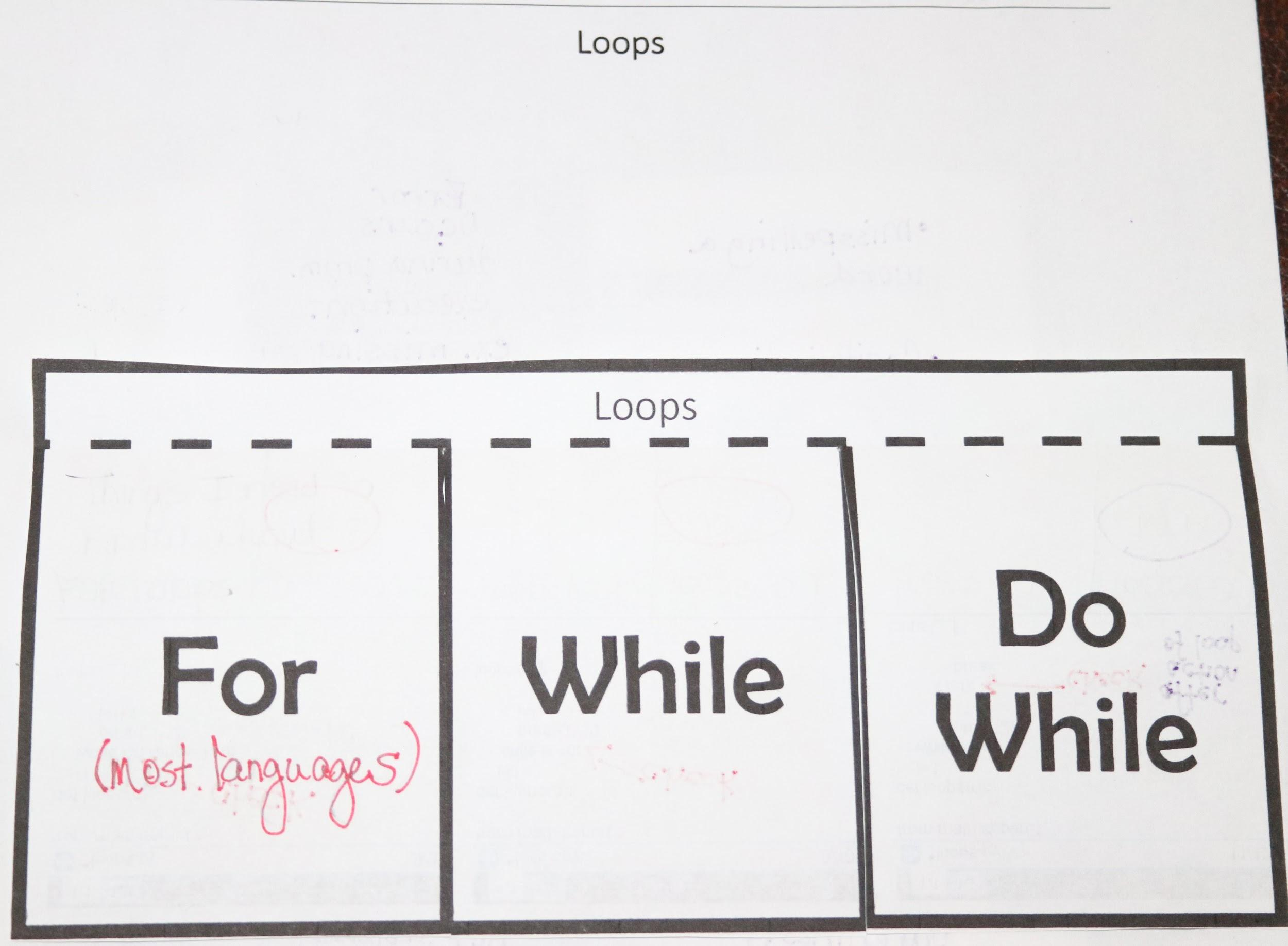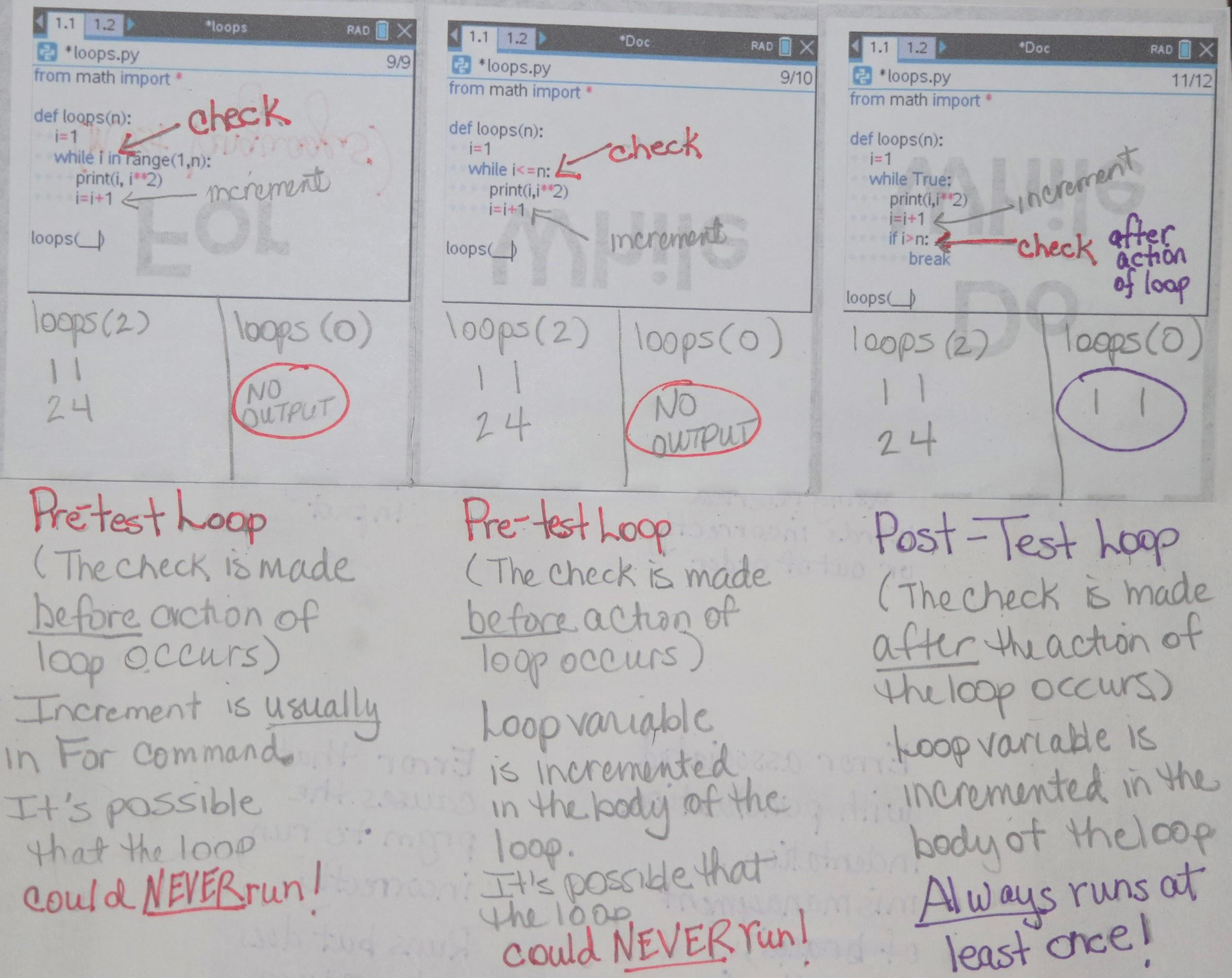## Incrementing variables

Consider the program shown below.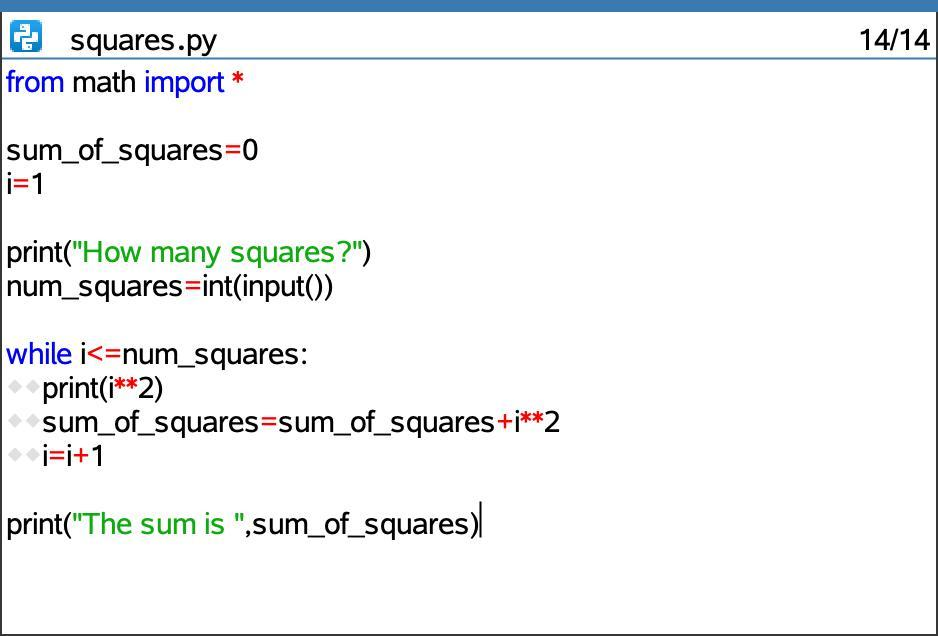How does this program work? Take a minute to explain the logic of this program aloud to yourself or a colleague.

This program starts by instantiating a variable sum_of_squares with a value of 0 and i with a value of 1.

sum_of_squares will act as an accumulator in this program, because it will be used to collectively store the cumulative value of the squares of a sequence of numbers. For example, for the sequence of the numbers {1, 2, 3, 4}, the variable sum_of_squares would have a value of 30 (12 + 22 + 32 + 42).

i acts as a counter in this program, because it will be used to keep track of how many numbers we’ve used so far or what number we are on.

The user is asked How many squares? and then this input is converted to an integer and stored in the variable num_squares.

So long as the user entered a number greater than or equal to 1, the program will then enter a While loop. Within this loop, the program will print the value of i2, then add this value to the sum_of_squares variable (accumulate), then add 1 to the counter variable i (count).

The While loop will continue to run (printing i2, accumulating the sum_of_squares and counting/incrementing i) until the value of i is greater than the value of num_squares.

Then, the program will printThe sum is ____.” and display the accumulated sum of the indicated number of squares.

The Incrementing Variables notes page contains this same program and annotates key learnings about counters and accumulators. Here are two pictures of this interactive notes page.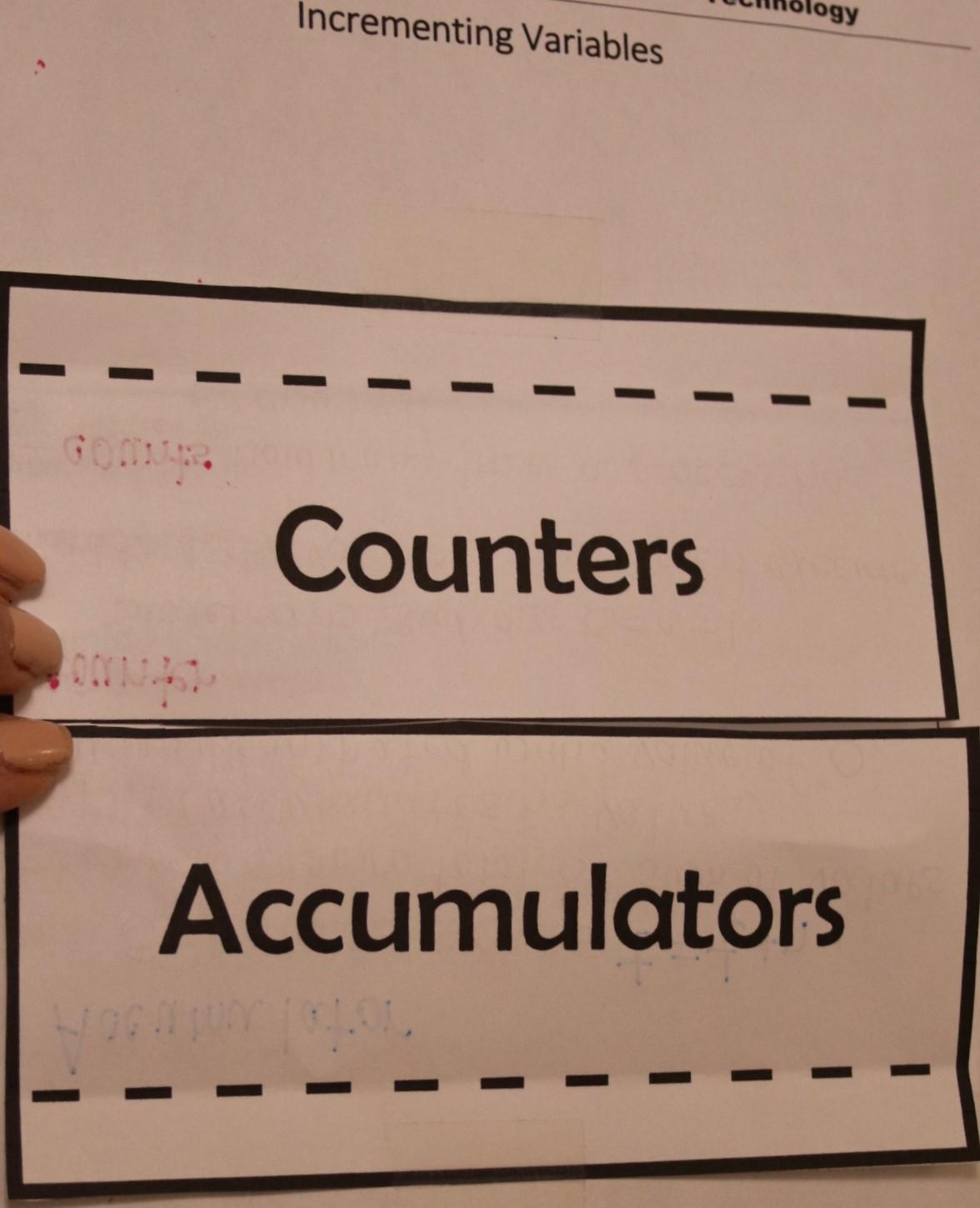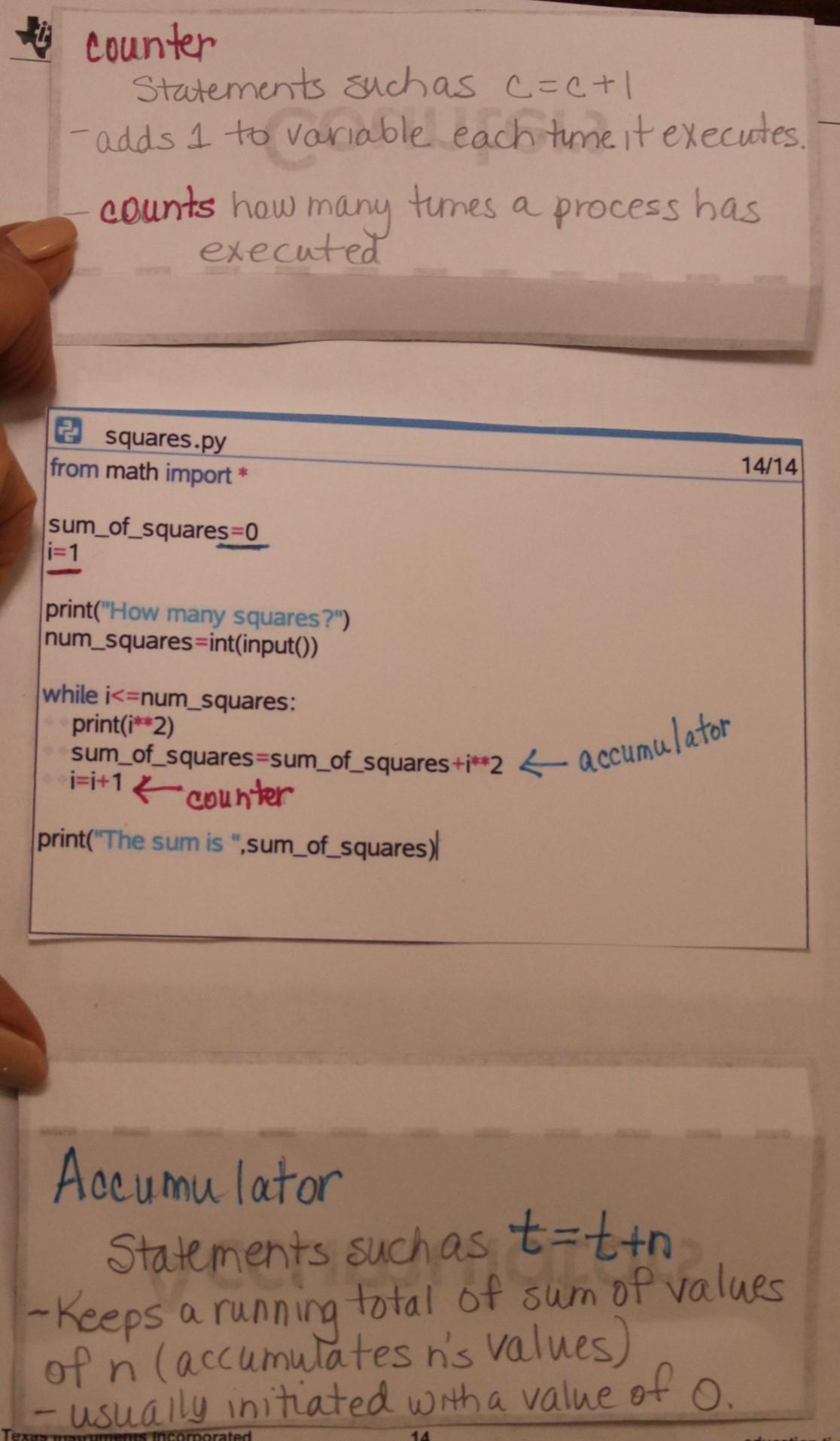## Unit 4 Application: Cube Root Game

Next, we’re going to apply what we’ve learned to write a program that operates like a game. Access the Unit 4, Application: Cube Root Game document and work through the application laid out there.

Here are some questions to think about as you get started on the task:

• What variables will you need to create? How will you use them?
• What kind of loop structure will you use for this program? Why?
• What will you display to the user and when? Why?

Once you’ve considered your responses to these questions, code out your game accordingly. Test it out. Does it work as you intended?

Here is one example of what the code for this game could look like. (There are many other ways to code a program that acts the same way.)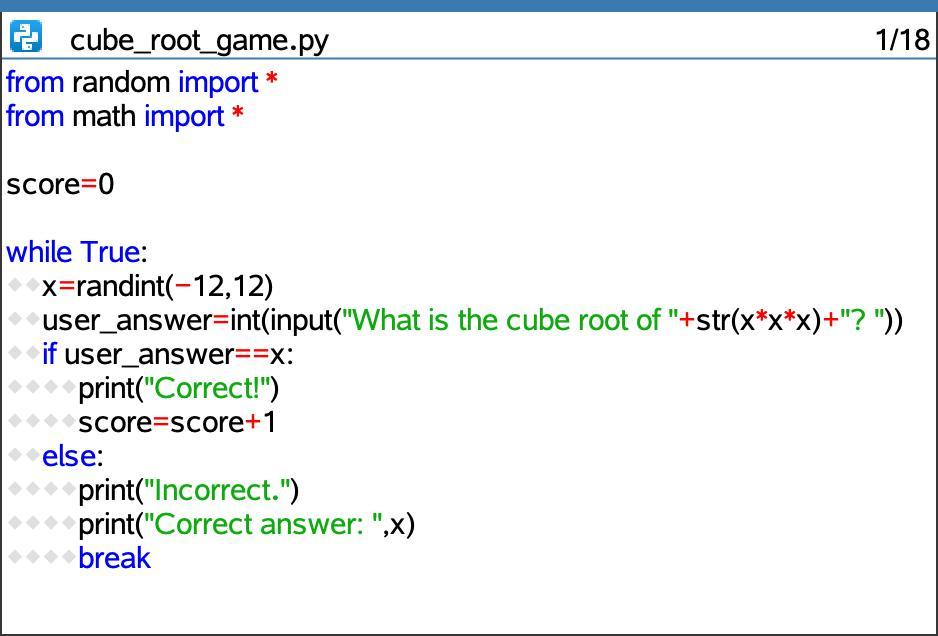This example program uses a Do While loop structure that will run at least one time. This allows for user input within the loop structure. The variable score is used as a counter that keeps track of how many questions the user answers correctly. The If-else structure returns different responses based on whether the user answers the question correctly or not. If the user answers incorrectly, the program tells them their answer was incorrect, displays the correct answer and then breaks the Do While loop and exits.

This sample program used a Do While loop. What could the program look like that uses a While loop with a conditional based on the user’s input? Would a For loop be a good fit for this type of program? Why or why not?

In what different ways could we format the print() outputs that the user sees?

What other enhancements can you add to this game?

## Reflecting on counters, accumulators, and loops

In this activity, we compared and contrasted the three types of loops (For, While and Do While), explored counters and accumulators, and applied our learning about these topics.

Consider:

• How are For, While and Do While loops similar? How are they different?
• What kinds of situations lend themselves to using a For loop? While loop? Do While loop?
• What are counters and accumulators? How are they used in programs?

The next activity in this series will explore another type of iteration: recursion.

This series includes: